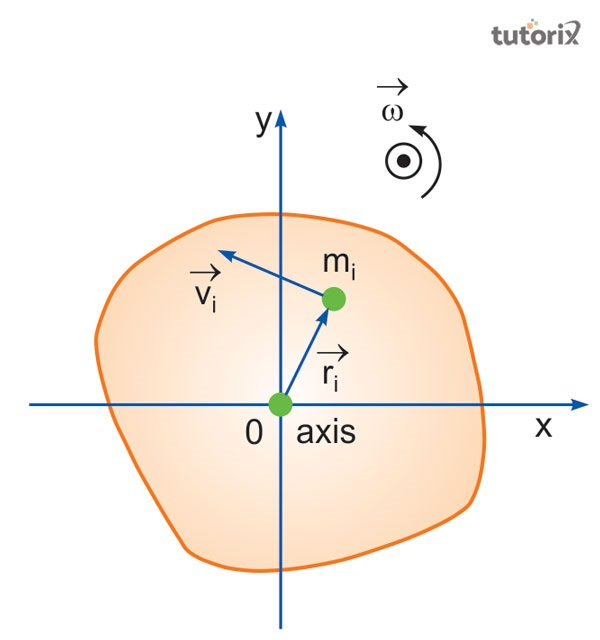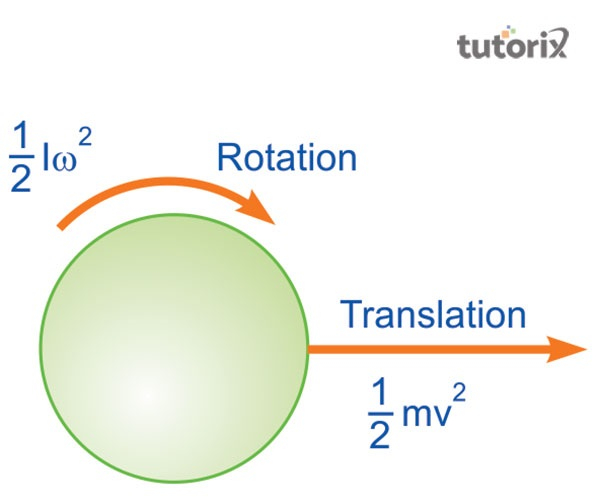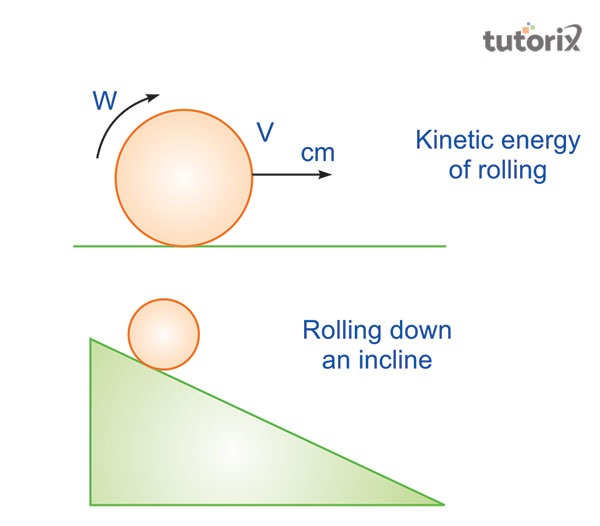# Rotational Kinetic Energy

## Introduction

The kinetic energy value of a centre of mass and of an extended object can be expressed with rotational kinetic energy. Both the linear and the rotational kinetic energy is developed based on the work-energy principle in a parallel manner. The representation of the rotational kinetic energy is done based on the particular axis of the object’s rotation.

## Rotational Kinetic energy: Analysis

During the time of an object’s rotation, rotational energy occurs. A significant part of total kinetic energy is named rotational energy. The moment of inertia occurs at the time when the rotational energy occurs separately based on an object’s rotation. Angular kinetic energy is another term that is used to define rotational energy (Cutting, Hindmarsh & Weir, 2020). A relevant and purposeful outcome of the rotation of an object is its rotational energy.Figure 1: Rotational kinetic energy

The relation between rotational kinetic energy and rotational inertia is proportional in nature. The square of the magnitude is also related to the angular velocity. Both the rotational kinetic energy and translational energy are present in a rolling object.

## The formula of rotational kinetic energy

A rotating object and its rotational energy can be measured by following a significant formula. In a mathematical way this formula can be presented as $\mathrm{K_R \:=\:1/2\: *I\: ω^{2}.}$ In this formula, $\mathrm{K_R}$ represents the value f rotational kinetic energy (Liu et al. 2018). The symbol ‘I’ denotes the value of the moment of inertia. ω in this formula represents the value of angular velocity.

The rotational kinetic energy is expressed by considering the half of the calculated product of the moment of inertia and angular velocity. Both the linear and rotational forms of kinetic energy can be expressed by this expression. The principle of work is proportional to the energy that is required for completing a whole rotation. The parallel nature between a moment of inertia and an exerted torque is associated with this (Zhao & Tresp, 2018). Force on a mass is denoted by m. This is considered from both the front and the end.

## Relation of Newton's Second Law with rotational kinetic energy

The concept of Newton’s second law is integrally connected to the base of rotational kinetic energy. As per Newton’s second law, the value of acceleration is equal to the resultant value of velocity. The value of velocity is to be divided by the exact amount of time.Figure 2: Rotational objective

`Newton’s second law of rotation is important as the value of angular acceleration is extracted from that. The average velocity value is extracted by considering half of the outcomes of the final velocity (Zhang et al. 2020). The axis of an object’s rotation varies from object to object. An unattached object and its rotational axis revolve around the center of mass.

## Relevant units of rotational Kinetic Energy

Rotational kinetic energy is expressed with J (Joules) in the SI unit system. In the MKS unit system, the unit is expressed by $\mathrm{kg.m^2.s^{-2}}$. In the dimensional kinetic formula, the rotational kinetic energy value is expressed by presenting this. The dimensional formula is also an important part that can be expressed with the basic formula of rotational kinetic energy. The dimensional formula, associated with a moment of inertia is equal to $\mathrm{M^1L^2T^0}$. The angular velocity is also associated with this (Ariska, Akhsan & Zulherman, 2018).

The dimensional formula of this is $\mathrm{M^0L^0T^{-1}}$. The value of rotational kinetic energy can be extracted by subtracting the value of angular velocity value and moment of inertia. The outcome of this subtraction is $\mathrm{M^1L^2T^{-2}}$.

## Characteristics of rotational kinetic energy

The kinetic energy is heavier in terms of any other object. The rotational kinetic energy can be transferred into other kinds of energy. There is a significant relationship between velocity and kinetic energy. This relation is proportional in nature. The kinetic energy is found to increase as well as decrease with the changes in an object’s velocity (Zhang et al. 2018). The rotational kinetic energy is measured and presented by using Joules. The direction of an object is integrally connected to the rotational kinetic energy. Usage of rotational kinetic energy helps the local users in using normal processes in generating energy.Figure 3: Relation between kinetic energy and rolling

## Conclusion

Rotational kinetic energy can be found in a windmill in which the blades move by the winds. The winds hit the blades and it rotates. This type of movement in the blades helps in generating electricity. Moving air possesses the kinetic energy in this term. In this process, kinetic energy is exported and converted into mechanical energy. The relation between work and energy can be analyzed based on this. In gravitation and uniform circular motion as well, the rotational kinetic energy holds an important impact.

## FAQs

Q1. What is the significance of the rotational power formula?

Ans. The rotational power formula is important as it helps in extracting the value of kinetic energy. Based on this formula, the value of the moment of inertia can also be calculated.

Q2. Which kinds of energies are present in a rolling object?

Ans. A rolling object is a great example of evaluating rotational kinetic energy. In such an object both the translational and the rotational kinetic energy are present.

Q3. What is the unit of measuring moments of inertia?

Ans. Unit of Magnetic Field is regarded as the suitable unit for measuring moments of inertia. The mass time and the distance are two important factors, associated with the measurement of moments of inertia.

Q4. Which factor affects the rotational kinetic energy of an object?

Ans. The speed of spinning of an object is the main factor on which the rotational kinetic energy is dependent. The faster the object spin, the energy level gets high. Location of the mass is also an important factor in this.

Updated on: 13-Oct-2022

185 Views